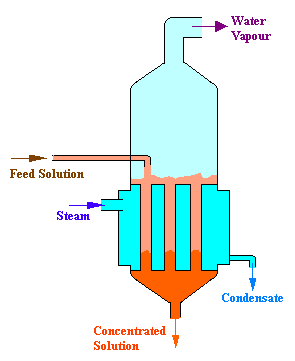### Steam Requirement for Evaporation

An evaporator is fed with10000 kg/hr of a solution containing 1% solute by weight. It is to be concentrated to 1.5% solute by weight. The feed is at a temperature of 37oC. The water is evaporated by heating with steam available at a pressure of 1.34 atm absolute, corresponding to a temperature of 108.3oC. The operating pressure in the vapor space is 1 atm absolute. Boiling point elevation and other effects can be neglected. The condensate leaves at the condensing temperature. All the physical properties of the solution may be taken to be same as that of water. What is the quantity of steam required per hour?

Data:
Enthalpy of feed = 38.1 kcal/kg
Enthalpy of solution inside the evaporator (at 100oC) = 98 kcal/kg
Enthalpy of vapor at 100oC = 644 kcal/kg
Latent heat of vaporization of steam = 540 kcal/kg

Calculations:Basis: 10000 kg/hr of solution containing 1% solute by weight.

Let us denote the feed stream as F and Concentrated solution stream as P, and water evaporated as W and the concentration of solute as x

Balance on solute:

Solute in the feed = solute in the concentrated liquor

i.e., Fx = Px

10000 x 0.01 = P x 0.015

therefore, P = 6666.7 kg/hr.

and water evaporated (W) = F - P = 10000 - 6666.7 = 3333.3 kg/hr.

Enthalpy balance:

FHF + Sλs = WHW + PHP

Where, HF = enthalpy of feed = 38.1 kcal/kg

HW = enthalpy of water vapor = 644 kcal/kg

HP = enthalpy of product = 98 kcal/kg

λs = Latent heat of steam = 540 kcal/kg

therefore,

10000 x 38.1 + S x 540 = 3333.3 x 644 + 6666.7 x 98

S = 4479.6 kg/hr

i.e., steam required = 4479.6 kg/hr.